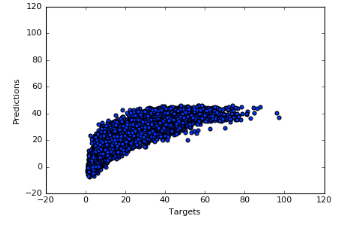# Feed Forward Neural Network Not Fitting Data

I have a very simple feed-forward neural network architecture set up to perform regression on a some what nonlinear dataset i.e the mapping from inputs (47 dimensional) to output (1 dimensional real value) is non-linear.

I wanted to start small and hence am trying to fit the data using the architecture described below. I am trying to replicate the architecture described in a publication wherein the only difference is the second-order Levenberg-Marquardt optimization method. However, I am seeing that my model is unable to handle the range of the target values and is only predicting in a small range. Any help with regard to explaining the current model behavior would be greatly appreciated.

To clarify, I have also included a histogram of the target values that are used to train the model (see below). From this, I guess the way I’m reading it is that the model is overfitting to the mean value of the distribution or some small range around the mean value? I have also tried SGD, Adam and RProp all yielding similar results as observed in this case. I also tried normalizing the target values to have a range between (-1,1) and even in that case, the training data scatter plot is similar to the one below. However, IMO, since I’m using a linear layer, the scale of the data shouldn’t really affect the model much and the model should be able to learn the range of y values but I could very well be wrong / missing something.

# Model

``````if torch.cuda.is_available():
device = torch.device('cuda')
else:
device = torch.device('cpu')

D_in  = X_train.shape
D_out = 1
H = 25
NUMEPOCHS = 400

# Fully connected neural network with one hidden layer
class NeuralNet(torch.nn.Module):
def __init__(self, input_size, hidden_size, output_size):
super(NeuralNet, self).__init__()
self.fc1  = torch.nn.Linear(input_size, hidden_size)
self.tanh = torch.nn.Tanh()
self.fc2  = torch.nn.Linear(hidden_size, output_size)

def forward(self, x):
out = self.fc1(x)
out = self.tanh(out)
out = self.fc2(out)

return out

model = NeuralNet(D_in, H, D_out).to(device)

# Loss and optimizer
criterion = torch.nn.MSELoss()
optimizer = optim.RMSprop(model.parameters(), lr=0.0001)

#Input Data
trainX = Variable(torch.from_numpy(X_train).float())
trainY = Variable(torch.from_numpy(y_train).float())
valX = Variable(torch.from_numpy(X_val).float())
valY = Variable(torch.from_numpy(y_val).float())
testX = Variable(torch.from_numpy(X_test).float())
testY = Variable(torch.from_numpy(y_test).float())

losses=list()

# Train the model
for epoch in range(NUMEPOCHS):
print("Epochs = {}".format(epoch))
alltargets=list()
allpredictions=list()

# Forward pass
outputs = model(batchX)
loss = criterion(outputs, batchY)
allpredictions.append(outputs)
alltargets.append(batchY)

# Backward and optimize
loss.backward()
optimizer.step()
losses.append(loss)
``````

Could you post the shape of `outputs` and `batchY` before passing it to the `criterion`?

I just figured out this was the problem literally 5 seconds ago when I got a friend to look at the code. The `outputs` tensor was n X 1 , and the `batchY` was a size n tensor. So I guess there was undesired broadcasting happening. @ptrblck. Could you suggest ways to account for this in the future? Maybe adding asserts before the loss function invocation requiring the two inputs to have the same size?

Also another question I had was , why the loss functions in pytorch already don’t do this internally and throw an error to avoid undesired broadcasting? Is there a use for this behavior?

Btw. here is the fit now. (still needs parameter / architecture tuning but it is much better than before)1 Like

Thanks for the feedback.
I was thinking which behavior would be better and based on the recent issues, I think I would prefer an error.
Awesome, you’ve figured it out!

Agreed. An error would definitely be better. Thanks for your help! @ptrblck.